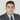# Kotlin and BIG Numbers

June 17, 2019

Very Big Numbers

## Introduction

In Java applications where financial integrity is crucial and no precision error can be admitted, Java developers have to use BigDecimal over Double or Float anytime.

To ease the life of developers for calculations and numbers exceeding Long.MAX_VALUE , the BigInteger object exists.

While being handy, using those two classes can quickly become unreadable for two main reasons :

• Lack of native operator : Java does not provide a way to simply operate two BigXXX objects without having to invoke a method on one of them. This adds unnecessary noise as you can see in the following example calculating the Pythagorean theorem

BigInteger a = BigInteger.valueOf(3); BigInteger b = BigInteger.valueOf(4); BigInteger c = BigInteger.valueOf(5); boolean isCorrect = a.pow(2).add(b.pow(2)).equals(c.pow(2));

• Immutability : the immutability of the objects forces the developers to manage the return values by assigning them back to the same reference, or a new one. This also adds unnecessary complexity

For those purposes, Kotlin proposes a new way to work with big numbers correcting the first problem, and by extension the second one is not that problematic anymore.

## Kotlin’s BigIntegers.kt and BigDecimals.kt

Those two classes are contained within the kotlin-stdlib-sources package and will certainly help improving readability, maintenance and testability of legacy our legacy code bases.

The simplicity comes in multilpe forms

• Overloaded operators : the ability to use binary and unary operators on BigXXX objects
• Extensions : the enrichment of the BigInteger and BigDecimal APIs with custom functions (e.g BigInteger.toBigDecimal() which doesn’t exist in the standard library)
• Infix functions : work the same way as overloaded operators but with names (e.g being able to bitwise operations using and , or or even xor )

Those 3 additions to the core features of the Kotlin language have made it possible to simplify the big numbers API for our everyday usage.

## Examples

Let’s take our initial example and simplify it to Kotlin

``````// Java
BigInteger a = BigInteger.valueOf(3);
BigInteger b = BigInteger.valueOf(4);
BigInteger c = BigInteger.valueOf(5);
boolean isCorrect = a.pow(2).add(b.pow(2)).equals(c.pow(2));

// Kotlin
val three = BigInteger.valueOf(3)
val four = BigInteger.valueOf(4)
val five = BigInteger.valueOf(5)
val isCorrect = (three *pow *2) + (four *pow *2) == (five *pow *2)``````

It is already more readable, but we don’t have the feeling to have a lot here.

Not that for this, I had to write a new infix function which is not included inside of the BigInteger class which is now reusable in my whole code base if I want to.

``public inline infix fun BigInteger.pow(power: Int): BigInteger = this.pow(power)``

Let’s now take a look at a second example.

There was before Java 9 no way to get the square root of a BigInteger using the API.

Developers were then forced to use custom implementations that were more or less precise. Let’s take one of them, rewrite it in Kotlin and use it as an extension function for our project.

The one we’ll be using is the accepted answer from this StackOverflow question.

This is what it looks like in Java

``````public static BigInteger sqrt(BigInteger x) {
BigInteger div = BigInteger.ZERO.setBit(x.bitLength()/2);
BigInteger div2 = div;
for(;;) {
BigInteger y = div.add(x.divide(div)).shiftRight(1);
if (y.equals(div) || y.equals(div2))
return y;
div2 = div;
div = y;
}
}``````

Let’s first convert it to plain Kotlin.

``````fun sqrt(bi: BigInteger): BigInteger {
var div = BigInteger.*ZERO*.setBit(bi.bitLength() / 2)
var div2 = div
while (true) {
when (val result = div + bi / div *shr *1) {
div, div2 -> return result
else -> {
div2 = div
div = result
}
}
}
}``````

It’s already far more readable.

Now let’s define it as an extension function of BigInteger and we’re set and done !

``````public inline fun BigInteger.sqrt(): BigInteger {
var div = BigInteger.*ZERO*.setBit(this.bitLength() / 2)
var div2 = div
while (true) {
when (val result = div + this / div *shr *1) {
div, div2 -> return result
else -> {
div2 = div
div = result
}
}
}
}``````

It required to change the argument list to not accept anything, the method signature to effectively be an extension of BigInteger and change the references to bi to be this instead.

Testing the function provides the correct results

``println(BigInteger.valueOf(2025).sqrt()) // Prints 45``

## Conclusion

One more reason to work with Kotlin as it will help you write more readable, maintainable and testable code even when using BigInteger or BigDecimal classes.Written by Yassin Hajaj , a passionate developer with interest in Java and Artificial Intelligence. You should follow them on LinkedIn